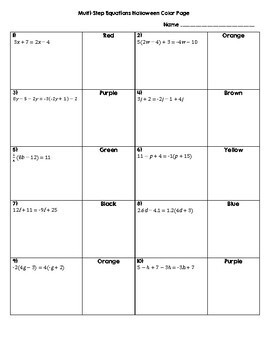# Multi-Step Equations Halloween Color Page Activity8th - 9th
Subjects
Standards
Resource Type
Formats Included
• PDF
Pages
5 pages

### Description

Add some engagement to your practice of multi-step equations with this Halloween color page activity! There are 10 equations for students to solve. Some include decimals, fractions, no solution, and infinitely many solutions. This is great practice for Common Core 8.EE.C7! I hope you and your students love this activity!

You may also like

Square Roots and Cube Roots Halloween Color Page

Systems of Equations Color Page

Pythagorean Theorem Scavenger Hunt

Total Pages
5 pages
Included
Teaching Duration
40 minutes
Report this Resource to TpT
Reported resources will be reviewed by our team. Report this resource to let us know if this resource violates TpT’s content guidelines.

### Standards

to see state-specific standards (only available in the US).
Solve linear equations with rational number coefficients, including equations whose solutions require expanding expressions using the distributive property and collecting like terms.
Give examples of linear equations in one variable with one solution, infinitely many solutions, or no solutions. Show which of these possibilities is the case by successively transforming the given equation into simpler forms, until an equivalent equation of the form 𝘹 = 𝘢, 𝘢 = 𝘢, or 𝘢 = 𝘣 results (where 𝘢 and 𝘣 are different numbers).
Solve linear equations in one variable.